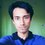# Proof Problem Of The Day - Digits and Sums!

For a positive integer $N$, let $S(N)$ be the sum of digits in the decimal representation of $N$. Let $F(N)$ be the sum of all the numbers obtained by erasing one or more numbers from the right end of $N$.

Prove that $S(N)+9F(N)=N$

Click here to see all the problems posted so far. Keep checking everyday!Note by Mursalin Habib
6 years, 10 months ago

This discussion board is a place to discuss our Daily Challenges and the math and science related to those challenges. Explanations are more than just a solution — they should explain the steps and thinking strategies that you used to obtain the solution. Comments should further the discussion of math and science.

When posting on Brilliant:

• Use the emojis to react to an explanation, whether you're congratulating a job well done , or just really confused .
• Ask specific questions about the challenge or the steps in somebody's explanation. Well-posed questions can add a lot to the discussion, but posting "I don't understand!" doesn't help anyone.
• Try to contribute something new to the discussion, whether it is an extension, generalization or other idea related to the challenge.
• Stay on topic — we're all here to learn more about math and science, not to hear about your favorite get-rich-quick scheme or current world events.

MarkdownAppears as
*italics* or _italics_ italics
**bold** or __bold__ bold
- bulleted- list
• bulleted
• list
1. numbered2. list
1. numbered
2. list
Note: you must add a full line of space before and after lists for them to show up correctly
paragraph 1paragraph 2

paragraph 1

paragraph 2

[example link](https://brilliant.org)example link
> This is a quote
This is a quote
    # I indented these lines
# 4 spaces, and now they show
# up as a code block.

print "hello world"
# I indented these lines
# 4 spaces, and now they show
# up as a code block.

print "hello world"
MathAppears as
Remember to wrap math in $$ ... $$ or $ ... $ to ensure proper formatting.
2 \times 3 $2 \times 3$
2^{34} $2^{34}$
a_{i-1} $a_{i-1}$
\frac{2}{3} $\frac{2}{3}$
\sqrt{2} $\sqrt{2}$
\sum_{i=1}^3 $\sum_{i=1}^3$
\sin \theta $\sin \theta$
\boxed{123} $\boxed{123}$

## Comments

Sort by:

Top Newest

Interesting.

- 6 years, 8 months ago

Log in to reply

At first it seems rather tedious. However, if we prove by induction on the number of digits the calculations will magically disappear!

When number of digits is 1, $F(N)=0, S(N)=n$, done.

Suppose it was true for all $k$ digit numbers. Consider a $(k+1)$ digit number $X$ with last digit $m$. Let $Y$ be the $k$ digit number without $m$. Then $X=10Y+m$. Hence $S(X)+9F(X)=S(Y)+m+9F(Y)+9Y$ (since we missed out $Y$ when calcualting $F(Y)$).

But by inductive hypothesis, $S(Y)+9F(Y)=Y$, hence $S(X)+9F(X)=Y+m+9Y=10Y+m=X$ and we are done.

- 6 years, 10 months ago

Log in to reply

Sorry, I did not see Mursalin's comment below. I just realized it was a repetition.

- 6 years, 10 months ago

Log in to reply

At first let $N=\displaystyle\sum_{k=0}^n a_k 10^k$. We have $S(N)=\displaystyle \sum_{k=0}^n a_k$. Now we calculate and simplify $F(N)$. A little observation is required to find the compact form of $F(N)$

$F(N) = \sum_{i=1}^n \sum_{j=i}^n a_j 10^{j-i}=\sum_{i=1}^{n} \sum_{j=0}^{i-1} a_{i} 10^j=\sum_{i=1}^n\left(a_{i}\sum_{j=0}^{i-1} 10^j\right)=\sum_{i=1}^n a_{i}\dfrac{10^i-1}{9}.$

The rest is straight. Using the last form of $F(N)$ we have

$9F(N)=\sum_{i=1}^n a_i\left(10^i -1\right)=\sum_{i=1}^n \left(a_i10^i-a_i\right)=\sum_{i=1}^n a_i10^i -\sum_{i=1}^n a_i =N-S(N)$

and the result follows. $\square$

NOTE: If you're wondering how I reformed the sum of $F(N)$ in the third step above, say $N=3265$. Then clearly

$F(3625)=326+32+3.$

This is the form of the first sum. Now break further into

\begin{aligned} F(3625) &=&326+32+3 \\ &=&(300+20+6)+(30+2)+3 \\ &=&(300+30+3)+(20+2)+6 \\ &=&3(10^2+10^1+10^0)+2(10^1+10^0)+6\cdot 10^0. \end{aligned}

Observe the number of occurrences of the powers of $10$ for the $n$th digit and generalize to get the second sum.

- 6 years, 10 months ago

Log in to reply

Great job!

Another way of doing it is inducting on the number of digits of $N$.

Clearly the statement is true for any one digit-number.

Assume that the statement is true for any $k$-digit number.

Now for any $(k+1)$- digit number $b$, let $b=10a+c$ where $a$ is a $k$-digit number and $c$ is a one-digit number.

Notice that, $S(b)=S(a)+c$ and $F(b)=F(a)+a$

So, $S(b)+9F(b)=S(a)+c+9F(a)+9a$.

From the induction hypothesis, $S(a)+9F(a)=a$. Substituting that, we get,

$S(b)+9F(b)=10a+c=b$.

So the result holds for all positive integers $N$.

- 6 years, 10 months ago

Log in to reply

BTW, someone's downvoting every damn comment he's passing by. -_-

- 6 years, 10 months ago

Log in to reply

I don't mind being downvoted.

- 6 years, 10 months ago

Log in to reply

Neither do I. But I do mind being spammed.

- 6 years, 10 months ago

Log in to reply

×

Problem Loading...

Note Loading...

Set Loading...